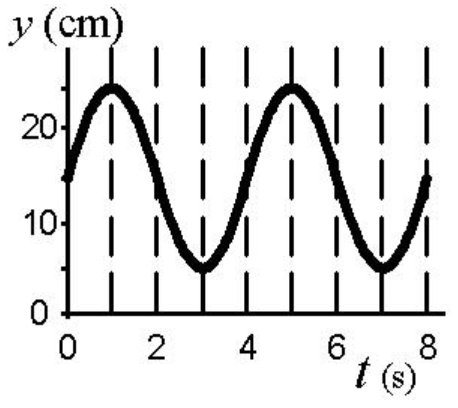# Problem: What is the maximum velocity of the object (in cm/s)?

###### FREE Expert Solution

The maximum velocity of a sinusoidal wave is given by:

$\overline{){{\mathbf{v}}}_{\mathbf{m}\mathbf{a}\mathbf{x}}{\mathbf{=}}{\mathbf{A}}{\mathbf{\omega }}}$

Frequency and periodic time:

$\overline{){\mathbf{f}}{\mathbf{=}}\frac{\mathbf{1}}{\mathbf{T}}}$

Angular frequency:

$\overline{){\mathbf{\omega }}{\mathbf{=}}{\mathbf{2}}{\mathbf{\pi }}{\mathbf{f}}}$

The time occupied by one complete wave (periodic time) is:

T = 4 s.

1 s-1 = 1 Hz

87% (478 ratings)###### Problem DetailsWhat is the maximum velocity of the object (in cm/s)?